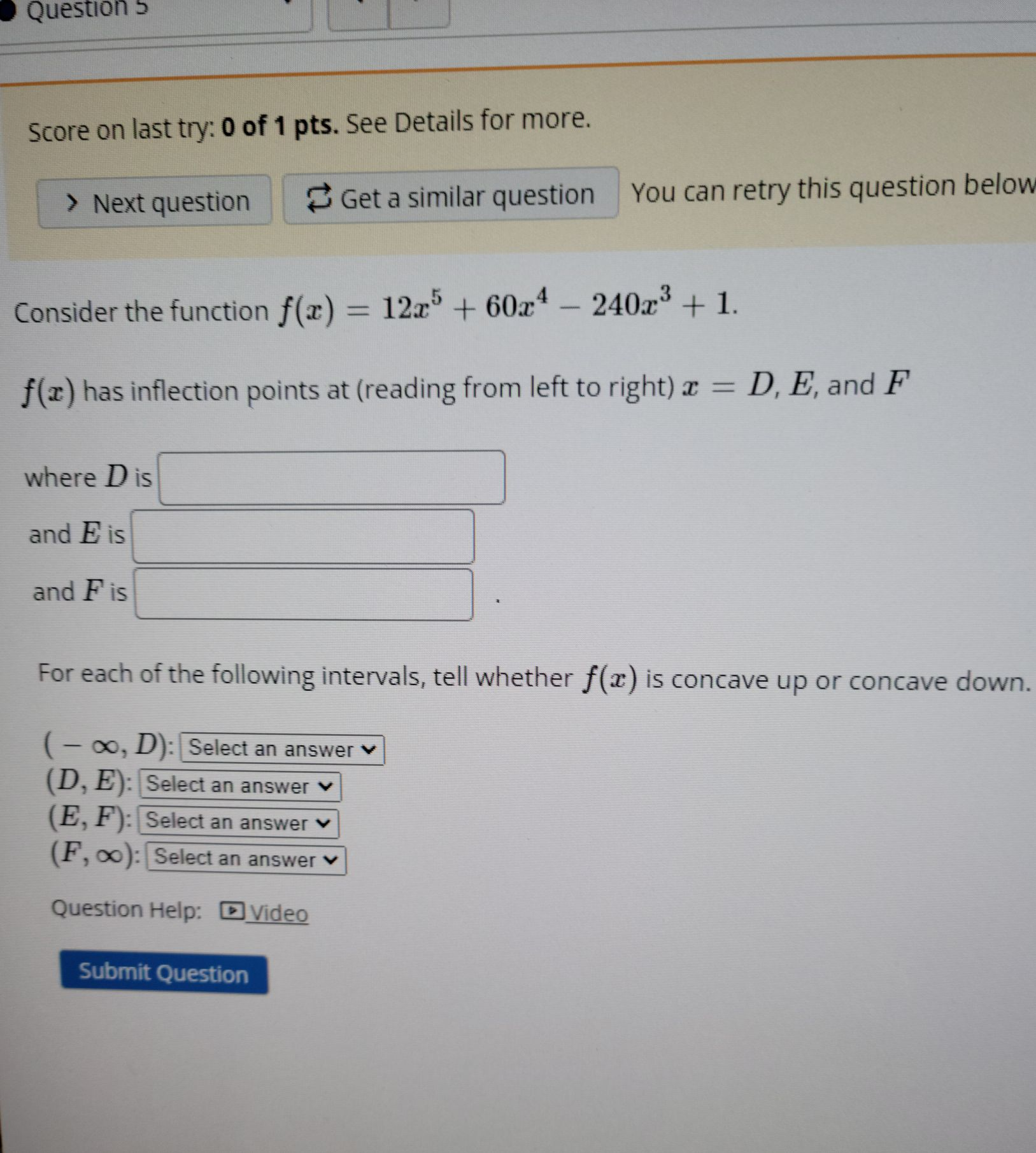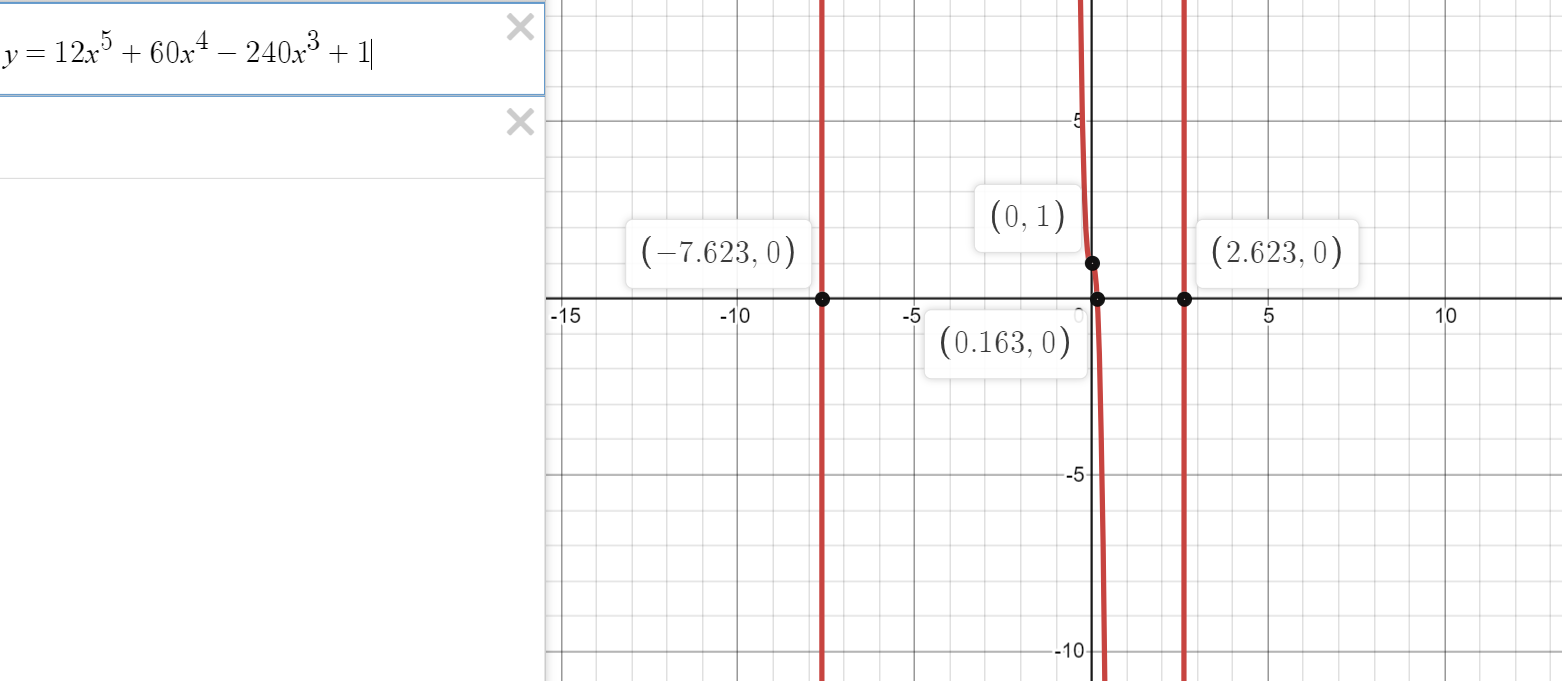### Still have math questions?

Algebra
QuestionConsider the function $$f ( x ) = 12 x ^ { 5 } + 60 x ^ { 4 } - 240 x ^ { 3 } + 1$$

$$f ( x )$$ has inflection points at (reading from left to right) $$x = D , E$$ , and $$F$$ where $$D$$ is and $$E$$ is and $$F$$ is For each of the following intervals, tell whether $$f ( x )$$ is concave up or concave down.

$$( - \infty , D )$$ : Select an answer $$\vee$$

$$( D , E )$$ : Select an answer $$\vee$$

$$( E , F ) :$$ Select an answer $$\vee$$

$$( F , \infty ) :$$ Select an answer $$\vee$$